# Geometric Solids Cylinders and Cones 1 Cylinders Cylinder

• Slides: 14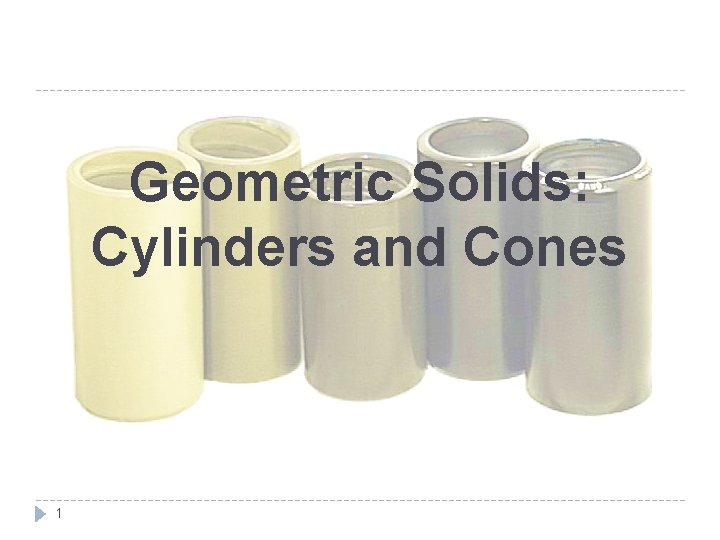Geometric Solids: Cylinders and Cones 1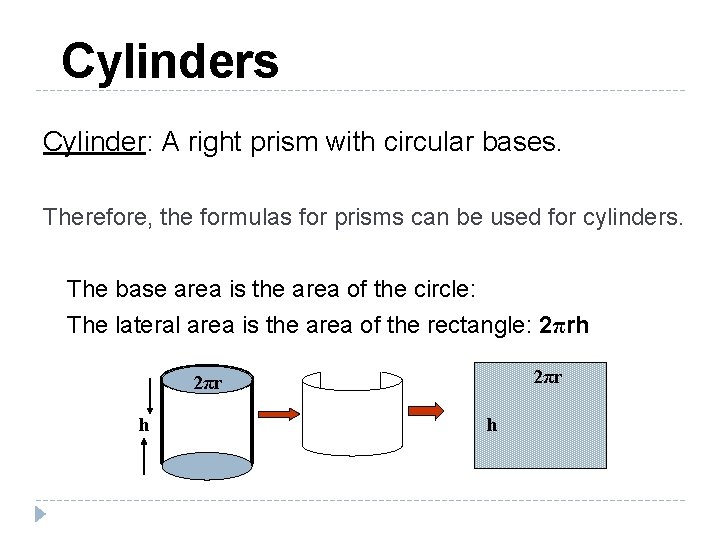Cylinders Cylinder: A right prism with circular bases. Therefore, the formulas for prisms can be used for cylinders. The base area is the area of the circle: The lateral area is the area of the rectangle: 2πrh 2πr h hFormulas for Cylinders Formulas: S. A. = 2πr ( r + h ) V= Surface Area (SA) = 2 B + LA = 2πr ( r + h ) Volume (V) = Bh = 2πr h hExample #1 4 cm For the cylinder shown, find the lateral area , surface area and volume. 3 cm V = πr 2 • h V = π(3)2 • (4) L. A. = 2πr • h L. A. = 2π(3) • (4) L. A. = 24π sq. cm. V = 36π S. A. = 2 • πr 2 + 2πr • h S. A. = 2 • π(3)2 + 2π(3) • (4) S. A. = 18π +24π S. A. = 42π sq. cm. 4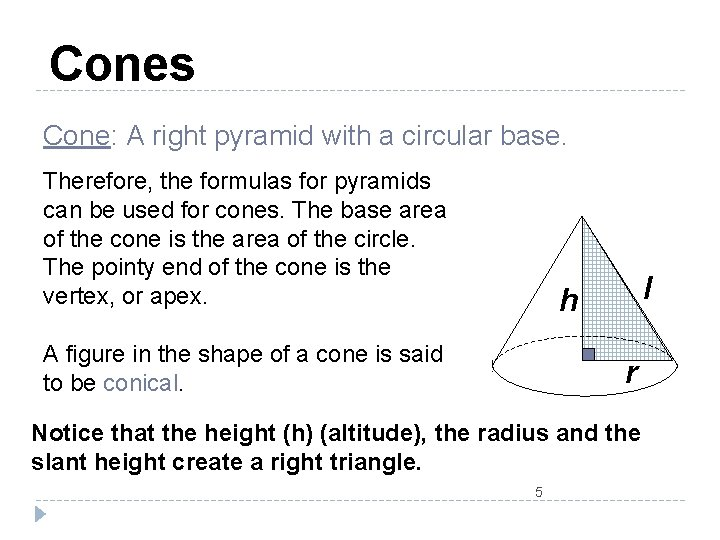Cones Cone: A right pyramid with a circular base. Therefore, the formulas for pyramids can be used for cones. The base area of the cone is the area of the circle. The pointy end of the cone is the vertex, or apex. l h A figure in the shape of a cone is said to be conical. r Notice that the height (h) (altitude), the radius and the slant height create a right triangle. 5Formulas for Cones Formulas: S. A. = πr ( r + l ) V= Lateral Area (LA) = π r l Where l is the slant height. Surface Area (SA) = π r ( r + l ) l h The base area is the area of the circle: r Volume (V) = 6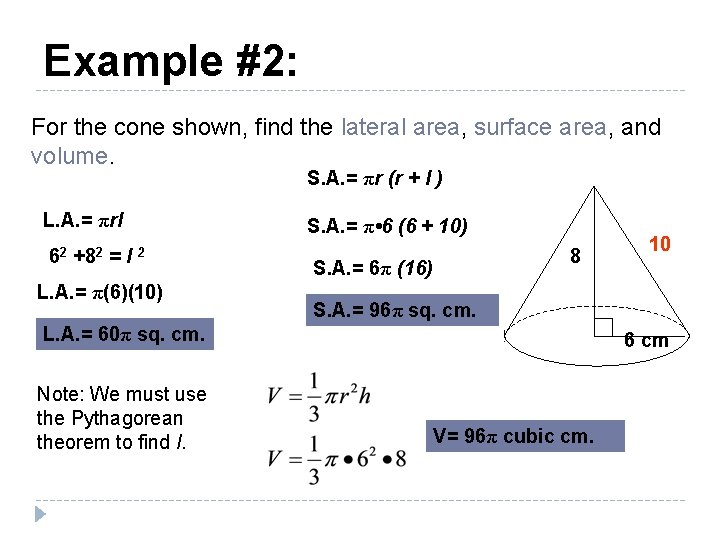Example #2: For the cone shown, find the lateral area, surface area, and volume. S. A. = πr (r + l ) L. A. = πrl 62 +82 = l 2 L. A. = π(6)(10) S. A. = π • 6 (6 + 10) 8 S. A. = 6π (16) S. A. = 96π sq. cm. L. A. = 60π sq. cm. Note: We must use the Pythagorean theorem to find l. 10 6 cm V= 96π cubic cm.Example #3: Find the volume of the cylinder with a radius of 4 inches and a height of 6 inches. Find the volume of the same cylinder with half the radius and the same height. How does the volume change? It is a quarter of the original volume! 8 inches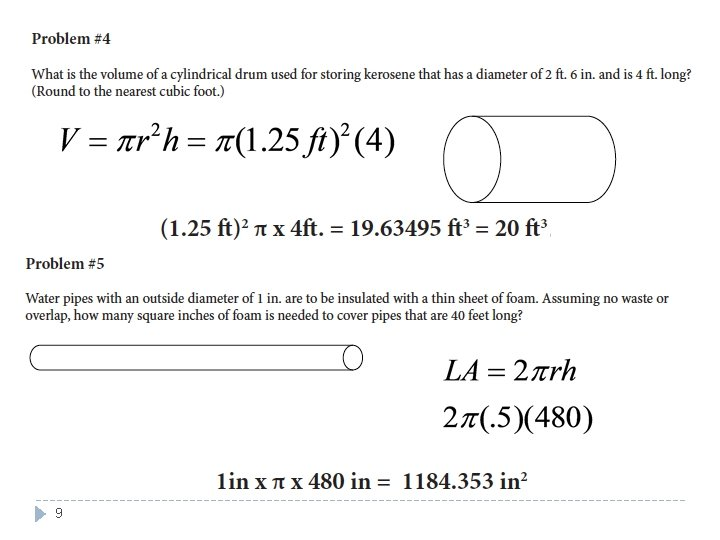9Example #6: r 4 ? h 3 2 l ? 6 L. A. ? ? r h l 4 3 5 2 6 10 T. A. ? ? L. A. V ? ? T. A. VExample #7 Guess which contains more? *Note, images not perfect OR drawn to scale. Option 1. A can of soda in the shape of a cylinder with a diameter of 5 cm and a height of 10 cm. 11 Option 2. A bottle of soda in the shape of a cylinder AND a cone, with a diameter of 4 cm. , a height of 10 cm. , and a cone height of 6 cm.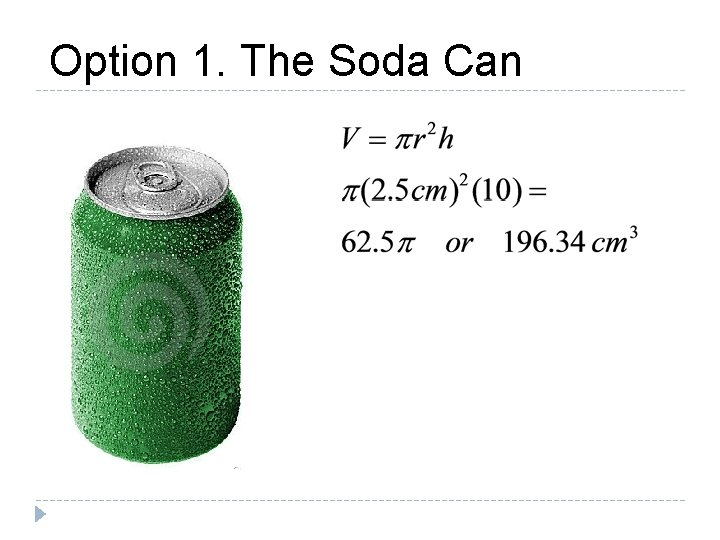Option 1. The Soda CanOption 2. The Bottle ** The bottle is a cylinder with a cone on top.The Winner!